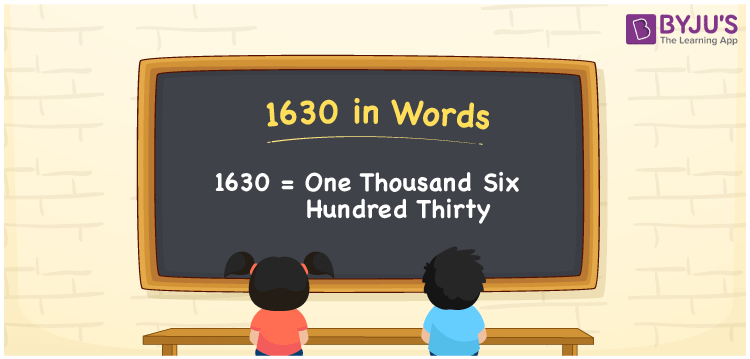# 1630 in Words

1630 in words is written as One thousand six hundred thirty. In both the International System of Numerals and the Indian System of Numerals, 1630 is written as One thousand six hundred thirty.

We often use 1630 as “1630 students in the assembly hall”. 1630 represents a quantity, “how much of something” or “how many of something”. Hence, the number 1630 is a Cardinal Number.

 1630 in Words One thousand six hundred thirty One thousand six hundred thirty in Number 1630

## 1630 in English Words

1630 in English words is read as “One thousand six hundred thirty.”## How to Write 1630 in Words?

To write 1630 in words, we shall use the place value chart. In the place value chart, put 1 in the thousands, 6 in the hundreds, 3 in the tens and 0 in the ones, respectively. Let us make a place value chart to write the number 1630 in words.

 Thousands Hundreds Tens Ones 1 6 3 0

Thus, we can write the expanded form as

1 × Thousand + 6 × Hundred + 3 × Ten + 0 × One

= 1 × 1000 + 6 × 100 + 3 × 10 + 0 × 1

= 1000 + 600 + 30 + 0

= 1630

= One thousand six hundred thirty.

1630 is a natural number which lies between1629 and 1631.

1630 in words – One thousand six hundred thirty

• Is 1630 an odd number? – No
• Is 1630 an even number? – Yes
• Is 1630 a perfect square number? – No
• Is 1630 a perfect cube number? – No
• Is 1630 a prime number? – No
• Is 1630 a composite number? – Yes

## Frequently Asked Questions on 1630 in Words

Q1

### How to write 1630 in words?

1630 in words is written as One thousand six hundred thirty.
Q2

### How to write 1630 in words in the International and Indian System of Numerals?

In both, the system of numerals, 1630 in words, is written as One thousand six hundred thirty.
Q3

### How to write 1630 in a place value chart?

In the place value chart, write 1 in the thousands, 6 in the hundreds, 3 in the tens and 0 in the ones, respectively.